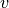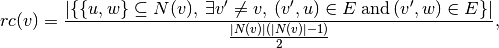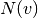Warning

This documents an unmaintained version of NetworkX. Please upgrade to a maintained version and see the current NetworkX documentation.

# node_redundancy¶

node_redundancy(G, nodes=None)[source]

Computes the node redundancy coefficients for the nodes in the bipartite graph G.

The redundancy coefficient of a nodeis the fraction of pairs of neighbors ofthat are both linked to other nodes. In a one-mode projection these nodes would be linked together even ifwere not there.

More formally, for any vertex, the redundancy coefficient of v is defined bywhereis the set of neighbors ofin G.

Parameters: G (graph) – A bipartite graph nodes (list or iterable (optional)) – Compute redundancy for these nodes. The default is all nodes in G. redundancy – A dictionary keyed by node with the node redundancy value. dictionary

Examples

Compute the redundancy coefficient of each node in a graph:

>>> import networkx as nx
>>> from networkx.algorithms import bipartite
>>> G = nx.cycle_graph(4)
>>> rc = bipartite.node_redundancy(G)
>>> rc
1.0


Compute the average redundancy for the graph:

>>> import networkx as nx
>>> from networkx.algorithms import bipartite
>>> G = nx.cycle_graph(4)
>>> rc = bipartite.node_redundancy(G)
>>> sum(rc.values()) / len(G)
1.0


Compute the average redundancy for a set of nodes:

>>> import networkx as nx
>>> from networkx.algorithms import bipartite
>>> G = nx.cycle_graph(4)
>>> rc = bipartite.node_redundancy(G)
>>> nodes = [0, 2]
>>> sum(rc[n] for n in nodes) / len(nodes)
1.0

Raises: NetworkXError – If any of the nodes in the graph (or in nodes, if specified) has (out-)degree less than two (which would result in division by zero, according to the definition of the redundancy coefficient).

References

  Latapy, Matthieu, Clémence Magnien, and Nathalie Del Vecchio (2008). Basic notions for the analysis of large two-mode networks. Social Networks 30(1), 31–48.